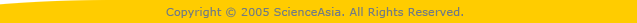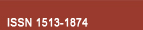| Home  | About ScienceAsia  | Publication charge  | Advertise with us  | Subscription for printed version  | Contact us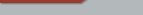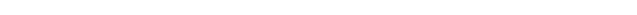Editorial Board Journal Policy Instructions for Authors Online submission Author Login Reviewer Login Volume 49 Number 5 Volume 49 Number 4 Volume 49 Number 3 Volume 49 Number 2 Volume 49 Number 1 Volume 48 Number 6 Earlier issues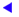Volume 44 Number 1Volume 44 Number 2 Volume 44 Number 3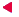previous article next article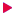8669

Research articles

ScienceAsia 44 (2018): 125-128 |doi: 10.2306/scienceasia1513-1874.2018.44.125

# OVD-characterization of simple K3-groups

## ShitianÿLiua,b, DonglinÿLeia, XianhuaÿLia,*

ABSTRACT:     A vanishing element of G is an element gG such that χ(g)=0 for some χ∈Irr(G). Let Van(G) denote the set of vanishing elements of G, i.e., Van(G)={gG | χ(g)=0forsomeχ∈Irr(G)}. We define vo(G) to be the set {o(g) | g∈Van(G)} consisting of the orders of the elements in Van(G), that is, vo(G)={o(g) | g∈Van(G)}. Obviously, vo(G)⊆ω(G) where ω(G) is the set of element orders of G. Let πV(G) be the set of prime divisors of the orders of the vanishing elements of G, that is, πV(G)={π(o(g)) | g∈Van(G)}. Obviously πV(G)⊆π(G) where π(G) denotes the set of the prime divisors of the order |G| of a group G. Let G be a finite group and |G|=p1α1p2α2...pkαkpk+1αk+1...pnαn, where the pi are different primes and the αi are positive integers. Assume that πV(G)={p1,p2,...,pk}. For p∈πV(G), let deg(p):=|{q∈πV(G)|pq}|, which we call the vanishing degree of p. We also define VD(G):=(deg(p1),deg(p2),...,deg(pk)), where p1<p2<...<pk. We call VD(G) the vanishing degree pattern of G. In this paper, we give a characterization of simple K3-groups by group orders and their vanishing degree patterns of the vanishing prime graphs.